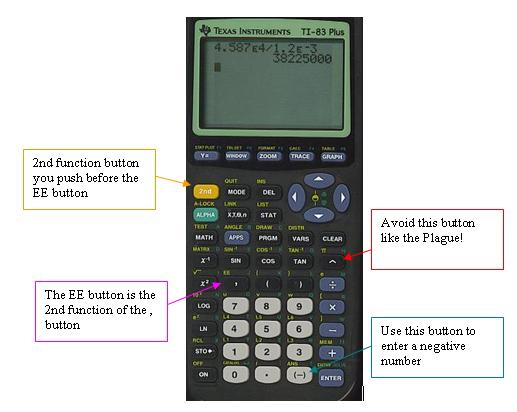# What are some examples of scientific notation on a scientific calculator?

Jul 19, 2014

Most scientific calculators express numbers like 2×10⁻³ as 2E-3.

The "E" stands for "Exponent" or "×10 to the…"

Consider the calculation

4.587 × 10⁴ ÷ 1.2 × 10⁻³ = 38 225 000 or 3.8225 × 10⁷

On a TI calculator, for example, you first press the 2nd function (2nd) key and then the Enter Exponent key (EE) to get the "E". Then you use the Change Sign key "(-)" to enter a negative number.Do not enter 2×10⁻³ by pressing 2, then the × button, then 10, then the ^ button, and then -3. Your calculator will treat this as two separate numbers. You will get some calculations wrong because it screws up the proper order of operations.

Always use the EE button.

Other things to avoid: Do not use the ${10}^{x}$ button to enter exponents. Do not use the subtraction sign to enter a negative exponent. Use the Change Sign key.

On other calculators the button may be labelled EXP (middle button in the bottom row in the image below). You may not have to press the 2nd function key first.On still other calculators, the Change Sign key is shown as "+/-".http://socratic.org/chemistry/useful-analysis-tips/correct-use-of-a-scientific-notation-on-a-scientific-calculator